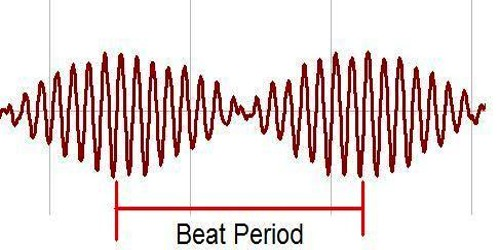# Mathematical Expression of Beat

Mathematical Expression of Beat

Let consider two sounded tuning forks having frequencies n1 and n2 (n1> n2) and the difference between the two frequencies is not large. Furthermore, let us consider that incoming sound waves from the two tuning forks after incident on a particle of the medium at same phase, the separate displacement of the particle after ‘t’ second due to the two waves are,

y1 = a sin 2π n1t …. … … (1)

and, y2 = a sin 2π n2t …. … … (2)

Resultant displacement due to superposition,

y = y1 + y2 = [a sin 2π n1t] + [a sin 2π n2t]

If the amplitudes of the two waves are same i.e., a = b, then,

y = a [sin 2π n1t + sin 2π n2t]

= [2a cos 2π (n1 – n2)t /2] . sin 2π [(n1 + n2)2]t

let, A = 2a cos 2π (n1 – n2)t /2] and, n = (n1 + n2)2

So, y = A sin 2πnt … … …. (3)

This equation, like equations (1) and (2), is the resultant equation of the wave. Its frequency is M and amplitude is A. This amplitude will be different with variation of time. This is because those two sound waves when meet on a particle their phase difference changes with time. At one instant the two waves of the particle meet at same phase and at other instant they meet at opposite phase. As a result, due to combined effect of the two waves, the displacement or intensity of sound at one time becomes very intense and at other time becomes feeble. Due to this periodic variation of sound beats are formed. For example-

If, t = 0, 1/(n1 – n2), 2/(n1 – n2), 3/(n1 – n2) etc. then amplitude A will be-

A = 2a, – 2a, 2a, – 2a etc.

So, at these times amplitude will be maximum and sound will be heard loudly. This is because that intensity of sound is proportional to the square of amplitude.

Again, if t = 1/ 2(n1 – n2), 3/ 2(n1 – n2), 5/ 2(n1 – n2) etc. then A = 0

So, at these moments, no sound will be heard. Hence it is seen that, between two intense sounds or between two feeble or zero sounds time interval, T = 1/(n1 – n2) and this  is the time period of decrease or increase of sound or beat.

So, number of beats in 1 second or frequency = 1/T = (n1 – n2) = difference of frequencies of the two sound waves.

Generally, it can be written, N = (n1 ~ n2)

According to this equation unit of beat will be “per second” or second-1.